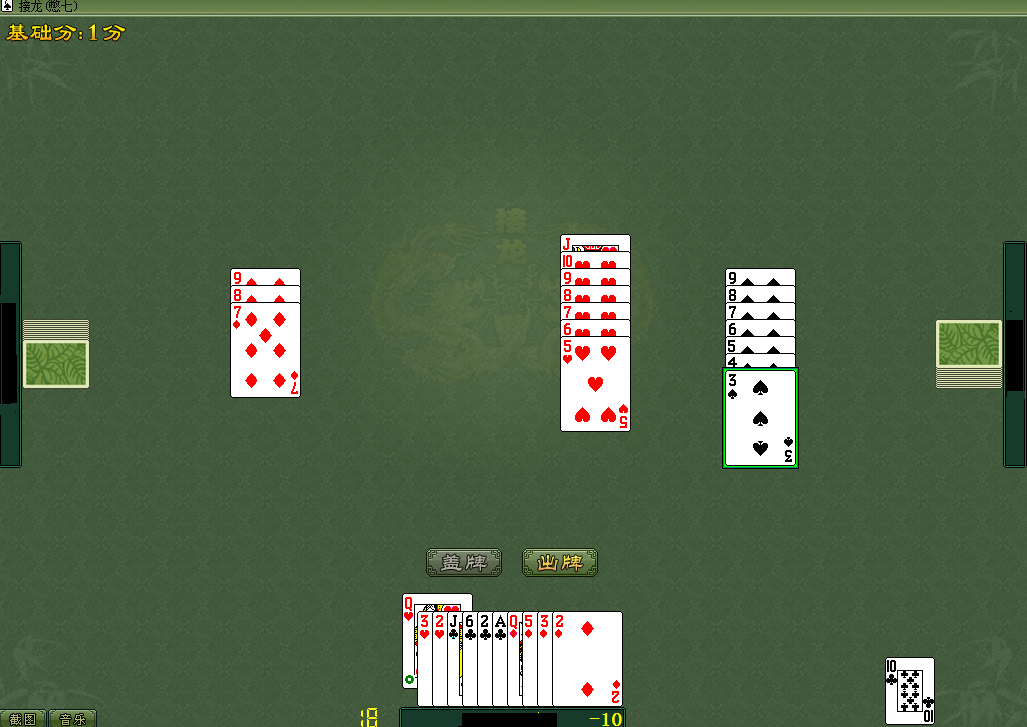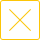# 憋七规则大揭秘A：5分；B：8分；C：1分；D：12分。

A的得分=(B扣牌分一A扣牌分)+(c扣牌分一A扣牌分)+(D扣牌分一A扣牌分)

B的得分=(A扣牌分一B扣牌分)+(c扣牌分一B扣牌分)+(D扣牌分一B扣牌分)

C的得分=(A扣牌分一C扣牌分)+(B扣牌分一C扣牌分)+(D扣牌分一C扣牌分)

D的得分：(A扣牌分一D扣牌分)+(B扣牌分一D扣牌分)+(C扣牌分一D扣牌分)

A：(8—5)+(1—5)+(12—5)=3—4+7：6分。

B：(5—8)+(1—8)+(12—8)=一3—7+4=一6分。

C：(5—1)+(8—1)+(12—1)=4+7+11=22。

D：(5—12)+(8—12)+(1—12)=一7—4—11=一22。

## 接龙(憋七)热门攻略更多